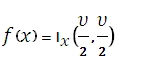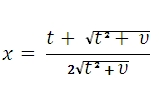# SQL Server T.DIST function

Updated: 9 August 2010

Use TDIST to calculate the probability for the Student t-distribution where a numeric value (x) is a calculated value of t for which the probability is to be computed. The t-distribution is used in the hypothesis testing of small sample data sets. Use this function in place of a table of critical values for the t-distribution. TDIST calculates the cumulative distribution function which is given by the incomplete beta function:WhereSyntax
SELECT [wctStatistics].[wct].[TDIST] (
<@X, float,>
,<@Degrees_freedom, float,>
,<@Tails, int,>)
Arguments
@X
is the value to at which to evaluate the distribution. @X is an expression of type float or of a type that can be implicitly converted to float
@Degrees_freedom
is a number specifying the degrees of freedom. @Degrees_freedom is an expression of type float or of a type that can be implicitly converted to float
@Tails
specifies the number of distribution tails to return.If @Tails = 1, TDIST returns the one-tailed distribution. If @Tails = 2, TDIST returns the two-tailed distribution. @Tails is an expression of type int or of a type that can be implicitly converted to int
Return Types
float
Remarks
·         If @Degrees_freedom  < 1, TDIST returns an error.
·         @Degrees_freedom is truncated to zero decimal places.
·         If @Tails is < 1 or @Tails > 2, TDIST returns an error.
·         If @X < 0, TDIST returns an error.
Examples

SELECT
wct.TDIST(1.959999998, 60, 1)

This produces the following result

----------------------
0.0273224648564988

(1 row(s) affected)

select

This produces the following result

----------------------
0.0273224648564988

(1 row(s) affected)

SELECT
wct.TDIST(1.959999998, 60, 2)

This produces the following result

----------------------
0.0546449297129976

(1 row(s) affected)

select
wct.FDIST(power(1.959999998,2),1,60) )

This produces the following result

----------------------
0.0546449297178828

(1 row(s) affected)

### SupportCopyright 2008-2023 Westclintech LLC         Privacy Policy        Terms of Service